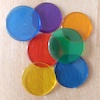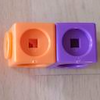#### You may also like### Nim-7

Can you work out how to win this game of Nim? Does it matter if you go first or second?### Break it Up!

In how many different ways can you break up a stick of 7 interlocking cubes? Now try with a stick of 8 cubes and a stick of 6 cubes.### Lots of Lollies

Frances and Rishi were given a bag of lollies. They shared them out evenly and had one left over. How many lollies could there have been in the bag?

# Next-door Numbers

##### Age 5 to 7Challenge Level
Magnus, Micah and Sam from Albyn in Aberdeen Scotland wrote:

We were not surprised when we realised that you always got an odd number because if you add an odd number and an even number it equals an odd number. When you are also doing the sum you find that it alternates between odd and even numbers as you go up the number line. So if you add 1+2 you will get an odd number which is 3.

From Monarch Global Academy Maryland in the USA, we had these three submissions:

Dylan
When you add next door numbers, like 13 and 14, you are adding an even and odd number.  When you add an even and odd number the sum will always be odd.
For example 13+14 = 27.
All next door numbers are odd plus even because when you count up it's a pattern.  Even, odd, even, odd.  So next door numbers will never be even plus even, or odd plus odd.
When you add an even and an even number, the sum will be even.  For example 8+8 = 16.
When you add two odd numbers, they will equal and an even number.  For example 13 + 17 = 30.

Kelly
All my answers are odd.  This did not surprise me because I'm adding an odd and an even number, so the sum will always be odd. When you add next door numbers you are always adding an odd and an even number because numbers go up even, odd, even, odd.
I figured out another pattern. If you count up and add each next door number your sum will always be four more than the last sum.

1+2 = 3, 3+4= 7 (4 more than 3), 5+6 = 11 (4 more than 7), 7+8 = 15 (4 more than 15).

Olivia and Charlotte
All our answers are odd because if you add an even and odd number your answers will always be odd.  If you add 10+11 it equals 21 because if you added 10+10 it would have been 20, but 10+11 is one more 21 which is odd.
If you add two even numbers the answers will always be even.  But when we add next door numbers we will never be adding even + even.  Next door numbers are always odd plus even or even plus odd.  For example 1+2 = 3.

Marcus from Woodland Primary School sent in the following:

Let the first number be n and second n+1.
The result will be n+n+1 which simplifies to 2n+1.
2n is an even number because it is a multiple of 2 and 1 more than any multiple of 2 is an odd number.
So, in this case, the answer will always be even.

Dylan from Middle School South sent in the following:

The thing I noticed was that the resulting number is always one more than the beginning number times two.
For example:
13+14=27
13 x 2 = 26
26 and 27 are one away.

The way to figure out why this happens is to set all numbers as a variation of a variable
A + A+1 = ?
A+1 is the next consecutive number from A and the question mark is the result.
When we add A and A+1 we get 2A+1,  2A+1 is one more than A doubled.
No matter what the starting number is, the next consecutive number added to it will result in two times the starting number plus 1.

Thank you for these solutions.  If you have any other ways to explain why we always get an odd number when we add two next-door numbers, then do let us know.  Is there a way to explain it by drawing a picture?